8th Grade Math Home > Teacher Resources > Activities > Human Coordinate Plane: Teacher Notes

Human Coordinate Plane: Teacher Notes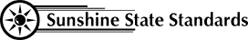MA.7.G.4.3
MA.8.A.1.1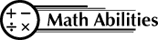Conceptual Knowledge

Coordinate Plane
Axis
Slope
Intercept

Procedural Knowledge

Graphing Coordinate Pairs
Representing Lines and Inequalities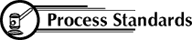Problem Solving
Reasoning
Communication
Connections
Representation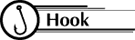Arrange 25 desks in a square array and have students sit in them. Explain that they are a human coordinate plane and each of them is to receive a card with an ordered pair (x,y) on it. Ask students who are not seated to distribute the ordered pair cards to the appropriate location. They may need to refer to a grid with points labeled as shown:

 (-2,2) (-1,2) (0,2) (1,2) (2,2) (-2,1) (-1,1) (0,1) (1,1) (2,1) (-2,0) (-1,0) (0,0) (1,0) (2,0) (-2,-1) (-1,-1) (0,-1) (1,-1) (2,-1) (-2,-2) (-1,-2) (0,-2) (1,-2) (2,-2)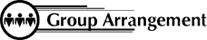Students work individually and as a class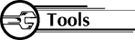• 25 large ordered pair cards labeled as shown above
• large grid with the points shown above labeled1. Ask the student whose ordered pair card has 0 as the first number to stand. Through discussion identify 0 as the x-coordinate and the students standing as the y-axis, they should now sit and students whose ordered card pair has 0 as the second number should stand. Again, discussion should identify the 0 as the y-coordinate and the students standing as the x-axis.

2. Ask each student with an x-coordinate of 1 to stand up and write x = 1 on the board. Now ask students with an x-coordinate of -2 to stand and write x = -2 on the board. Through discussion, lead students to see that equations of the form shown are:
1. a vertical line
2. parallel to the y-axis

3. Ask each student with a y-coordinate of 1 to stand up and write y = 1 on the board. Now ask students with a y-coordinate of -1 to stand and write y = -1 on the board. Through discussion, lead students to see that equations of the form shown are:
1. a horizontal line
2. parallel to the x-axis

4. Ask the students whose ordered pair has a sum of 1 to stand and write x + y = 1. These students should remain standing while students whose ordered pair first number - the second number equals 1 stand. Write x - y = 1 on the board. Through discussion, lead student to see that (1,0) is a point on both lines and represents the point of intersection. Substitute values in the equations on the board to show that (1,0) makes both x + y = 1 and x - y = 1 true.

5. Repeat the above process using x + y = 1 and x + y = 2. Guide students to discover that if there is no point of intersection, the lines are parallel.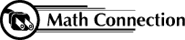As a result of this activity, students will have a better understanding of the coordinate plane.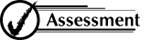Ask students whose ordered pair sum is 2 to raise their hands. Now ask students whose ordered pair sum in less than 2 to stand and write x + y < 2 on the board. Show the students a graph with a dotted line for x+ y = 2 and shading for x + y < 2. Note that the shading includes all points, not just integral values. Repeat the process for other inequalities.

 8th Grade Math Home > Teacher Resources > Activities > Human Coordinate Plane: Teacher Notes